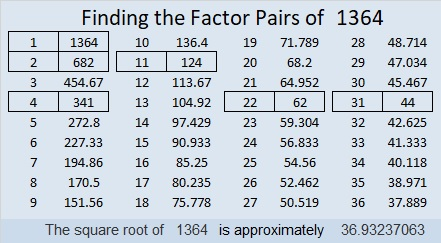# 1364 is the 15th Lucas Number

OEIS.org reminded me that the 15th Lucas Number is 1364, so I’ve made a graphic illustrating that fact:In this 40-second video I explain how to generate that list in excel using the drag feature:

Here are a few more facts about the number 1364:

• 1364 is a composite number.
• Prime factorization: 1364 = 2 × 2 × 11 × 31, which can be written 1364 = 2² × 11 × 31
• 1364 has at least one exponent greater than 1 in its prime factorization so √1364 can be simplified. Taking the factor pair from the factor pair table below with the largest square number factor, we get √1364 = (√4)(√341) = 2√341
• The exponents in the prime factorization are 2, 1, and 1. Adding one to each exponent and multiplying we get (2 + 1)(1 + 1)(1 + 1) = 3 × 2 × 2 = 12. Therefore 1364 has exactly 12 factors.
• The factors of 1364 are outlined with their factor pair partners in the graphic below.This site uses Akismet to reduce spam. Learn how your comment data is processed.